# Linear regression in Excel – continued

Doing linear regression in Excel is simple. Now I’d like to show you how exactly this is done.

Let’s use the same data set:

 x 9.42 2.78 5.87 5.49 6.48 4.91 7.82 2.7 2.65 8.42 5.85 0.93 y 52.24 4.15 13.72 7 5.28 6.98 42 7.15 3.41 31 12.59 1.09

Let’s say we want do a order-2 linear regression. That means, we want a function:

f(x)=c*x^2 + b*x+a

a, b and c should be chosen in a way such that if we put x into f(x), the difference/error between f(x) and y, will be minimized, in the least square sense.

So we have the difference R as a function of a,b,c：

R(a,b,c)=(c* 9.42^2 + b* 9.42 + a – 52.24)^2+(c*2.78^2+b*2.78+a-4.15)^2+…+(c*0.93^2+b*0.93+a)

R is smooth every where in space. So R is minimal where:

D(R)/D(a)=0; D(R)/D(b)=0; D(R)/D(c)=0

Now we’ll have to employ the compact form to avoid tedious typing: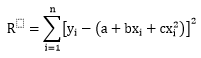In order for R to be minimal, we need: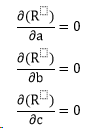Insert R into the equations and expand them, we get: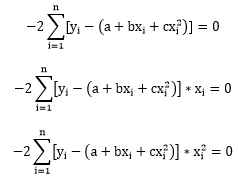That is: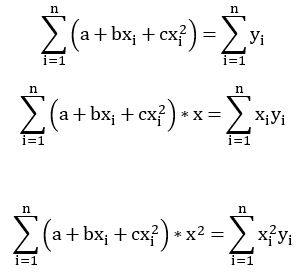Or in matrix form: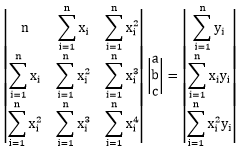Now this is a very simple equation, so we can simply solve it using Cramer’s rule:It looks scary but actually not too complex. We need the sum of x, x^2, x^3, x^4, x*y and x^y. We can actually verify this in excel:

 x y x^2 x^3 x^4 x*y x^2*y 9.42 52.24 88.69578 835.323 7866.942 491.974 4633.334 2.78 4.15 7.721688 21.45697 59.62447 11.53932 32.06537 5.87 13.72 34.43254 202.0476 1185.6 80.52797 472.5321 5.49 7.00 30.18894 165.8715 911.3719 38.47401 211.3934 6.48 5.28 41.99053 272.099 1763.204 34.19838 221.6059 4.91 6.98 24.08858 118.227 580.2595 34.23907 168.0457 7.82 42.00 61.22442 479.0568 3748.43 328.6334 2571.426 2.70 7.15 7.269068 19.59829 52.83935 19.27266 51.96143 2.65 3.41 7.003793 18.53532 49.05312 9.028243 23.89296 8.42 31.00 70.90802 597.0945 5027.948 261.0414 2198.149 5.85 12.59 34.27254 200.6409 1174.607 73.71933 431.5732 0.93 1.09 0.869064 0.810173 0.755272 1.014426 0.945685 63.32 186.61 408.665 2930.761 22420.63 1383.662 11016.92

So we have: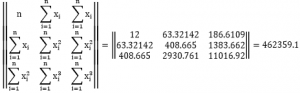So in the end we get: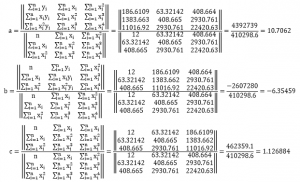This is exactly what Excel displayed on the chart: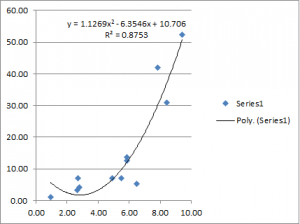I actually put all this in an Excel file so you can try it yourself. Please note that in the excel file we have a data set of 12 points. If your data set has a different size, you may have to adapt the formulas a little bit. 🙂